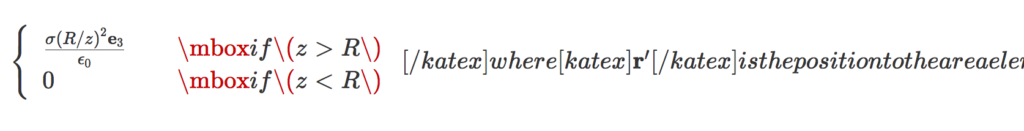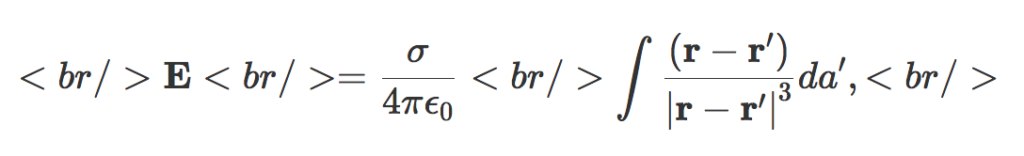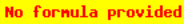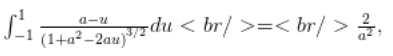## Attack took out my godaddy hosted wordpress blog for most of a day.Guilty admission: The title of this post is click-baity, as the attack was not likely on my blog, but something colocated on the server that my blog happened to have been hosted on.  In particular, the math, physics, complaining about COBOL, rants, and other random garbage that you’ll find on this blog does not likely warrant a DOS attack.  This isn’t the story of my offending somebody enough to get DOSed, but is just the story of a painful interaction with godaddy customer support.

I used to use a wordpress hosted blog, and eventually decided that I wanted flexibility enough to pay for hosting.  I experimented a bit with amazon hosting, but the variability in price scared me off, and I ended up buying my hosting from godaddy.  I don’t remember anymore what other options I considered, nor why I ended up settling on godaddy’s “managed wordpress” offering, over any others, although low initial cost was a factor.  That hosting has generally been problem free, but their IT support, when there is trouble, has proven to be less than desirable.  Here’s that story in case anybody else is considering using godaddy for their own hosting.

Yesterday, I happened to notice that my blog was completely unresponsive.  I only noticed this because I wanted to make one small change to one of my pages.  All told, to get this resolved, I spent about 3 hrs with their IT support (1/2 last night, and the other 1/2 today).  Ironically, by the time I got to the fifth support professional, the problem resolved itself.  I am glad that I don’t run any sort of business off of this site, as the downtime was at least 16 hours.

My 1.5 hrs on the godaddy IT chat support with Parjeet, Jaspreet, and Shibin was a complete waste of time.  Parjeet (who’s name I am probably butchering, since I didn’t keep a copy of my chat log with him) managed to get the blog restarted.  However, it appears that he also disabled all the plugins at the same time without telling me.  He also didn’t identify the root cause.  Jaspreet insisted that the issue was the content I was hosting, even though that content was not an issue before yesterday.  He gave me various self help options (plugin tuning, …) despite the fact that the blog was performing abysmally even with all plugins disabled, and had been okay prior to the reboot, and despite the fact that even the admin pages were slow, which have nothing to do with the content being served for normal blog page or post content.  He also was not able to identify the root cause, and I insisted on dealing with his manager at that point.  That claimed-manager was Shibin, who was helpful seeming, but was not able to do anything, nor able to find somebody who had access to the server logs to diagnose the issue.  When I gave up for the night, he promised to email me the results of his investigation, but no such email materialized.

The godadday response to an attack is pretty deficient.  If the server that your blog is running on is attacked, they throttle the performance of that server to mitigate the effectiveness of the attack.  The idea is that the attacker will eventually just give up.  That is done apparently done at the server level, and not just for the instance that is under attack.  It seems pretty dumb that godaddy doesn’t migrate the VMs that happen to be unfortunately colocated with attackee onto another physical host.  That’s not a good sign for anybody that wants a service that requires continuous uptime.

When I bought godaddy’s hosting initially, I do remember that it was one of the most cost effective options.  The godaddy hosting price went up considerably sometime after the first or second year of initial service, but I haven’t taken the time to figure out how to migrate to something else.  Perhaps amazon is worth looking at again? Basically, I’m allowing myself to be exploited financially a bit because the time cost to figure out how to migrate to other hosting is probably higher than the monetary cost of the blog hosting itself.

The support interaction that I had over the last two days might be enough of a kick in the butt that I’ll take the time to look at other hosting options, and how to do a migration.  One thing that I do recall was nice about amazon was they offered ssh access to the machine.  I only get sftp access on godaddy, which can be a pain in the butt, and is very inflexible.

You might wonder why I even bothered switching from wordpress.com hosting, which was free.  I did that to have the flexibility to install my own non-wordpress.com sanctioned plugins.  For somebody who is crazy enough to blog a lot of mathematics, that was very worthwhile, as I’ve been able to run a customized version of the Mathjax-Latex plugin, which renders very nicely, and allows me to replicate many of the latex macros that I use.  That streamlines my latex-to-wordpress conversion considerably, and has saved me many many hours.  That saving is in comparison to the time that would have been required to blog the same mathematics with the default wordpress.com latex plugin.  Recently, I also installed the Mathematica Toolbox plugin, which looks like it will allow some fun interactivity, much like the original Wolfram CDF plugin had before it became useless and eventually was no longer supported (i.e. it only worked in 32-bit browsers.)  So, I don’t think that I’m going to go back to wordpress.com hosting, but it’s definitely worth some investigation of the options.

## Electric field of a spherical shell. Ka-Tex rendered [Take II].

In a previous post I attempted to use the katex plugin to render an old post instead of using Mathjax. It seems that was not actually rendered with KaTex, but (I think) it was rendered with the latex keyword handling in the Jetpack plugin, which I also had installed. I’ve customized the katex plugin I have installed to use a different keyword (katex instead of latex).

This is a test of KaTex, the latex rendering engine used for Khan academy. They advertise themselves as much faster than mathjax, but this speed comes with some usability issues.

Here’s a rerendering of an old post, with the latex rendered with WP-KaTeX instead of MathJax-LaTeX.

# The post

### Problem:

Calculate the field due to a spherical shell. The field is

[katex display=”true”]\mathbf{E} = \frac{\sigma}{4 \pi \epsilon_0} \int \frac{(\mathbf{r} – \mathbf{r}’)}{{{\left\lvert{{\mathbf{r} – \mathbf{r}’}}\right\rvert}}^3} da’,[/katex]

where [katex]\mathbf{r}'[/katex] is the position to the area element on the shell. For the test position, let [katex]\mathbf{r} = z \mathbf{e}_3[/katex].

### Solution:

We need to parameterize the area integral. A complex-number like geometric algebra representation works nicely.

[katex display=”true”]\begin{aligned}\mathbf{r}’ &= R \left( \sin\theta \cos\phi, \sin\theta \sin\phi, \cos\theta \right) \\ &= R \left( \mathbf{e}_1 \sin\theta \left( \cos\phi + \mathbf{e}_1 \mathbf{e}_2 \sin\phi \right) + \mathbf{e}_3 \cos\theta \right) \\ &= R \left( \mathbf{e}_1 \sin\theta e^{i\phi} + \mathbf{e}_3 \cos\theta \right).\end{aligned}[/katex]

Here [katex]i = \mathbf{e}_1 \mathbf{e}_2[/katex] has been used to represent to horizontal rotation plane.

The difference in position between the test vector and area-element is

[katex display=”true”]\mathbf{r} – \mathbf{r}’ = \mathbf{e}_3 {\left({ z – R \cos\theta }\right)} – R \mathbf{e}_1 \sin\theta e^{i \phi},[/katex]

with an absolute squared length of

[katex display=”true”]\begin{aligned}{{\left\lvert{{\mathbf{r} – \mathbf{r}’ }}\right\rvert}}^2 &= {\left({ z – R \cos\theta }\right)}^2 + R^2 \sin^2\theta \\ &= z^2 + R^2 – 2 z R \cos\theta.\end{aligned}[/katex]

As a side note, this is a kind of fun way to prove the old “cosine-law” identity. With that done, the field integral can now be expressed explicitly

[katex display=”true”]\begin{aligned} \mathbf{E} &= \frac{\sigma}{4 \pi \epsilon_0} \int_{\phi = 0}^{2\pi} \int_{\theta = 0}^\pi R^2 \sin\theta d\theta d\phi \frac{\mathbf{e}_3 {\left({ z – R \cos\theta }\right)} – R \mathbf{e}_1 \sin\theta e^{i \phi}} { {\left({z^2 + R^2 – 2 z R \cos\theta}\right)}^{3/2} } \\ &= \frac{2 \pi R^2 \sigma \mathbf{e}_3}{4 \pi \epsilon_0} \int_{\theta = 0}^\pi \sin\theta d\theta \frac{z – R \cos\theta} { {\left({z^2 + R^2 – 2 z R \cos\theta}\right)}^{3/2} } \\ &= \frac{2 \pi R^2 \sigma \mathbf{e}_3}{4 \pi \epsilon_0} \int_{\theta = 0}^\pi \sin\theta d\theta \frac{ R( z/R – \cos\theta) } { (R^2)^{3/2} {\left({ (z/R)^2 + 1 – 2 (z/R) \cos\theta}\right)}^{3/2} } \\ &= \frac{\sigma \mathbf{e}_3}{2 \epsilon_0} \int_{u = -1}^{1} du \frac{ z/R – u} { {\left({1 + (z/R)^2 – 2 (z/R) u}\right)}^{3/2} }. \end{aligned}[/katex]

Observe that all the azimuthal contributions get killed. We expect that due to the symmetry of the problem. We are left with an integral that submits to Mathematica, but doesn’t look fun to attempt manually. Specifically

[katex display=”true”]\int_{-1}^1 \frac{a-u}{{\left({1 + a^2 – 2 a u}\right)}^{3/2}} du = \frac{2}{a^2},[/katex]

if [katex]a > 1[/katex], and zero otherwise, so

[katex display=”true”]\boxed{ \mathbf{E} = \frac{\sigma (R/z)^2 \mathbf{e}_3}{\epsilon_0} }[/katex]

for [katex]z > R[/katex], and zero otherwise.

In the problem, it is pointed out to be careful of the sign when evaluating [katex]\sqrt{ R^2 + z^2 – 2 R z }[/katex], however, I don’t see where that is even useful?

# KaTex commentary

1. Conditional patterns, such as:

\left\{
\begin{array}{l l}
\frac{\sigma (R/z)^2 \mathbf{e}_3}{\epsilon_0}
& \quad \mbox{if $z > R$ } \\
0 & \quad \mbox{if $z < R$ }
\end{array}
\right.


messed up KaTex, resulting in render errors like:Using $$...$$ within math mode instead of $...$ also messed things up. Example:

\left\{
\begin{array}{l l}
\frac{\sigma (R/z)^2 \mathbf{e}_3}{\epsilon_0}
& \quad \mbox{if $z > R$ } \\
0 & \quad \mbox{if $z < R$ }
\end{array}
\right.


This resulted in a messed up parse like so:It looks like it's the mbox that messes things up, and not the array itself, so \text could probably be used instead.

2. The latex has to be all in one line, or else KaTex renders the newlines explicitly. Example:Having to condense all my latex onto a single line is one of the reasons I switched from the default wordpress latex engine to mathjax. It was annoying enough that I started paying for my wordpress hosting, and stopped posting on my old free peeterjoot.wordpress.com blog. Using KaTex and having to go back to single line latex would suck!

3. The rendering looks great, just like mathjax.
4. The Mathjax-Latex wordpress plugin has some support for equation labeling and references. I don't see a way to do those with the WP-KaTex plugin.
5. I can have a large set of macros installed in my default.js matching a subset of what I have in my .sty files. I don't see a way to do that with the WP-KaTex plugin, but perhaps there is just no documented mechanism. KaTex itself does have a macro mechanism.
6. The display isn't left justified like the wordpress latex, and looks decent.

## Electric field of a spherical shell. Ka-Tex rendered

This is a test of KaTex, the latex rendering engine used for Khan academy. They advertise themselves as much faster than mathjax, but it looks like the reason for that is because they generate images that look crappy unless the browser resolution is matched to the images just right.

Here’s a rerendering of an old post, with the latex rendered with WP-KaTeX instead of MathJax-LaTeX.

# The post

### Problem:

Calculate the field due to a spherical shell. The field is$\mathbf{E} = \frac{\sigma}{4 \pi \epsilon_0} \int \frac{(\mathbf{r} - \mathbf{r}')}{{{\left\lvert{{\mathbf{r} - \mathbf{r}'}}\right\rvert}}^3} da',$

where$\mathbf{r}'$ is the position to the area element on the shell. For the test position, let$\mathbf{r} = z \mathbf{e}_3$.

### Solution:

We need to parameterize the area integral. A complex-number like geometric algebra representation works nicely.\begin{aligned}\mathbf{r}' &= R \left( \sin\theta \cos\phi, \sin\theta \sin\phi, \cos\theta \right) \\ &= R \left( \mathbf{e}_1 \sin\theta \left( \cos\phi + \mathbf{e}_1 \mathbf{e}_2 \sin\phi \right) + \mathbf{e}_3 \cos\theta \right) \\ &= R \left( \mathbf{e}_1 \sin\theta e^{i\phi} + \mathbf{e}_3 \cos\theta \right).\end{aligned}

Here$i = \mathbf{e}_1 \mathbf{e}_2$ has been used to represent to horizontal rotation plane.

The difference in position between the test vector and area-element is$\mathbf{r} - \mathbf{r}' = \mathbf{e}_3 {\left({ z - R \cos\theta }\right)} - R \mathbf{e}_1 \sin\theta e^{i \phi},$

with an absolute squared length of\begin{aligned}{{\left\lvert{{\mathbf{r} - \mathbf{r}' }}\right\rvert}}^2 &= {\left({ z - R \cos\theta }\right)}^2 + R^2 \sin^2\theta \\ &= z^2 + R^2 - 2 z R \cos\theta.\end{aligned}

As a side note, this is a kind of fun way to prove the old “cosine-law” identity. With that done, the field integral can now be expressed explicitly\begin{aligned} \mathbf{E} &= \frac{\sigma}{4 \pi \epsilon_0} \int_{\phi = 0}^{2\pi} \int_{\theta = 0}^\pi R^2 \sin\theta d\theta d\phi \frac{\mathbf{e}_3 {\left({ z - R \cos\theta }\right)} - R \mathbf{e}_1 \sin\theta e^{i \phi}} { {\left({z^2 + R^2 - 2 z R \cos\theta}\right)}^{3/2} } \\ &= \frac{2 \pi R^2 \sigma \mathbf{e}_3}{4 \pi \epsilon_0} \int_{\theta = 0}^\pi \sin\theta d\theta \frac{z - R \cos\theta} { {\left({z^2 + R^2 - 2 z R \cos\theta}\right)}^{3/2} } \\ &= \frac{2 \pi R^2 \sigma \mathbf{e}_3}{4 \pi \epsilon_0} \int_{\theta = 0}^\pi \sin\theta d\theta \frac{ R( z/R - \cos\theta) } { (R^2)^{3/2} {\left({ (z/R)^2 + 1 - 2 (z/R) \cos\theta}\right)}^{3/2} } \\ &= \frac{\sigma \mathbf{e}_3}{2 \epsilon_0} \int_{u = -1}^{1} du \frac{ z/R - u} { {\left({1 + (z/R)^2 - 2 (z/R) u}\right)}^{3/2} }. \end{aligned}

Observe that all the azimuthal contributions get killed. We expect that due to the symmetry of the problem. We are left with an integral that submits to Mathematica, but doesn’t look fun to attempt manually. Specifically$\int_{-1}^1 \frac{a-u}{{\left({1 + a^2 - 2 a u}\right)}^{3/2}} du = \frac{2}{a^2},$

if$a > 1$, and zero otherwise, so$\boxed{ \mathbf{E} = \frac{\sigma (R/z)^2 \mathbf{e}_3}{\epsilon_0} }$

for$z > R$, and zero otherwise.

In the problem, it is pointed out to be careful of the sign when evaluating$\sqrt{ R^2 + z^2 - 2 R z }$, however, I don’t see where that is even useful?

# KaTex commentary

1. Conditional patterns, such as:

\left\{
\begin{array}{l l}
\frac{\sigma (R/z)^2 \mathbf{e}_3}{\epsilon_0}
& \quad \mbox{if $$z > R$$ } \\
0 & \quad \mbox{if $$z < R$$ }
\end{array}
\right.


messed up KaTex, resulting in render errors like:2. The latex has to be all in one line, or else KaTex renders the newlines explicitly. Example:Having to condense all my latex onto a single line is one of the reasons I switched from the default wordpress latex engine to mathjax. It was annoying enough that I started paying for my wordpress hosting, and stopped posting on my old free peeterjoot.wordpress.com blog. Using KaTex and having to go back to single line latex would suck!
3. The rendering looks like crap, unless you match your resolution to exactly those used to create the images. The mathjax rendering may be slower, but looks much better!
4. The Mathjax-Latex wordpress plugin has some support for equation labeling and references. I don’t see a way to do those with the WP-KaTex plugin.
5. I can have a large set of macros installed in my default.js matching a subset of what I have in my .sty files. I don’t see a way to do that with the WP-KaTex plugin, but perhaps there is just no documented mechanism. KaTex itself does have a macro mechanism.
6. Left justified display mode is hard to read. The mathjax rendered centered display mode looks much better.

## EDIT.

I’m not sure I was getting the katex plugin when I used the [ latex ] … [ /latex ] tags.  I see some comments that indicate that there is built in handling of these tags in the Jetpack plugin.  If I change frontend.php in the katex plugin to use [ katex ] … [ /katex ] tags instead, then I see much different results.

## Peeter Joot’s new blog: more to come…

After 611 blog posts on my old wordpress.com hosted blog, dating all the way back to 2009, I’ve decided to ante-up and pay for more flexible hosting.

My primary motivation for this was truly geeky. I wanted the flexibility to be able to manage wordpress plugins (i.e. mathjax-latex and wolframcdf), and to also be able to put plain old html and arbitrary file content into the apache2 directory structure. I’ve wanted plain html hosting for a while, but made do with google sites (i.e. crappy but free). I’d also wanted to be able to use the wolfram CDF plugin on my blog, but also not enough to pay for it. However, once I tried mathjax-latex, I was sold. Compared to wp-latex, this “new way” completely kicks ass, and should save me a lot of time.

I tried out an amazon EC2 bitnami image for a while (amazon offers a free trial year to evaluate their offerings). That’s a flexible setup and offers direct access to the Linux VM, which is very nice. However, with an amazon EC2 image, I’m not really sure what I would end up paying. The charts seem somewhat vague, depending on future usage of both machine and storage. I would also have pay separately for a domain name, and pay separately for amazon hosting of the DNS entry.

I ended up deciding to use a go-daddy hosted wordpress instance, which is a flat rate service. It is less flexible than a godaddy standalone web-hosting environment, but also cheaper ($12 for the first year, including the domain name, and ~$50/year after that). It also looks like I can upgrade this to a more generic web hosting environment later if the cost of that seems justified. I’ll see first if only having sftp access to htdocs is enough of a major inconvenience to pay that additional yearly fee. If not, then I may consider changing to another host.

Configuring a custom MathJax configuration was a bit of a pain with only sftp access, mostly because I had to copy the MathJax tree, which was very slow for so many small files. I did that directory tree transfer with FileZilla since sftp ‘put –r’ appears to be busted. This MathJax setup was way easier on the EC2 since the ssh shell allowed for wget and local unzip directly from the apache2 htdocs tree. It’s a shame that the mathjax-latex plugin doesn’t allow the MathJax tree to be served from the default server (what the plugin settings calls the ‘MathJax CDN Service’). Logically, I’d like to be able to use that CDN service, but have my configuration file hosted locally. That config file (config/default.js) is a single small file, and is likely all that I’ll ever have to alter in that whole directory tree.

I haven’t decided whether or not I’ll keep my old peeterjoot.wordpress.com blog, or switch unconditionally to this new peeterjoot.com blog (which will be the new home for any of my mathematical or physics related posts). This new blog has no blog-article content so far, and doesn’t yet have a theme template that I like. What is here so far is:

• An enumeration of things I have written, including archives of all the individual pdfs that I have posted over the years along with my blog entries. All these pdfs are now stored directly on the new site in the htdocs tree. I will no longer be using any of my (three) old google sites pages as pdf stores.
• A chronological listing of all the Mathematica notebooks I have written. The newest versions of these notebooks can still be found in my Mathematica github repository. A snapshot of each of these is now also available on the new site, so if you have the CDF plugin installed, these can now be examined by clicking on the links directly. Ironically, with chrome and my CDF installation, I’m able to view the .nb suffixed notebooks directly in the browser, but a click on any CDF (.cdf) notebook triggers a download?
• I’ve made a couple notes about my setup of the mathjax-latex plugin, and the differences in latex markup with that plugin compared to the wp-latex plugin (which is available by default on wordpress.com). My future mathematical blogging should be way easier, probably won’t require any of my old tex2blog script, and will also look better!
• An About page, copied directly from the About page on my old blog.

More to come, … now that I’ve finally finished the Stokes theorem chapter in my Geometric Algebra compilation, I expect new posts to be more frequent.